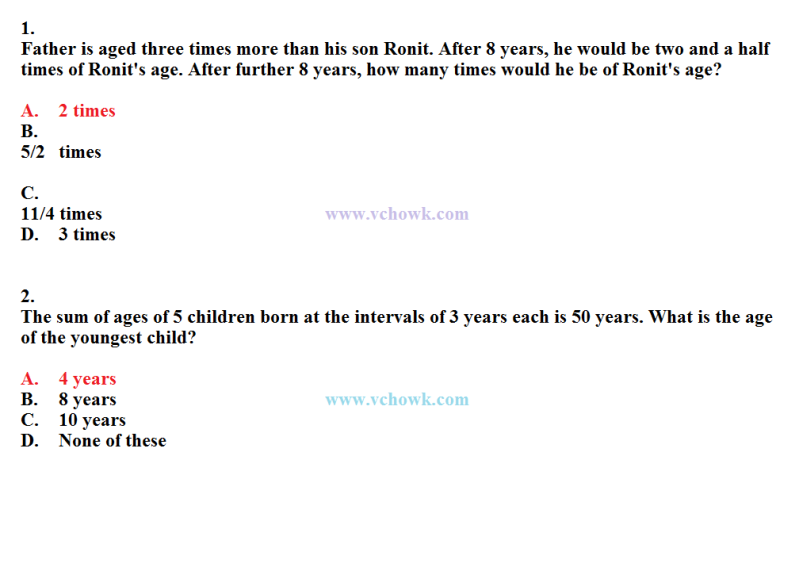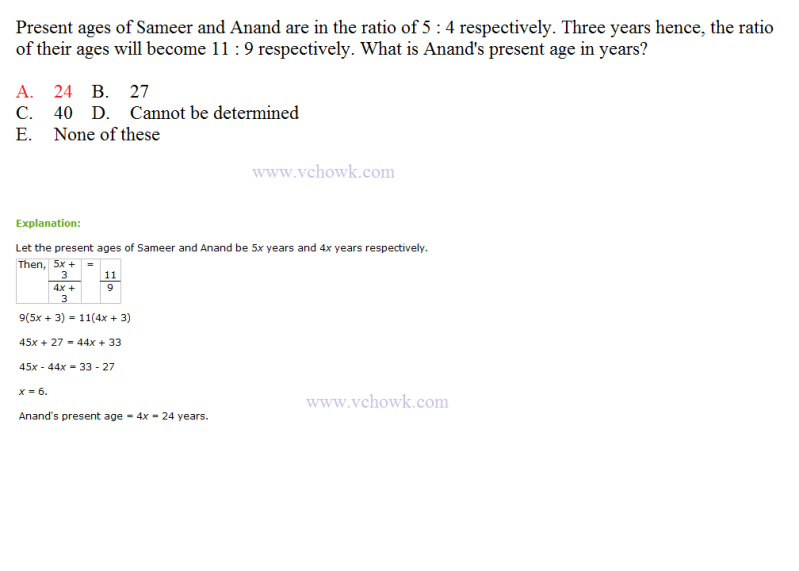## Aptitude > Problems on Ages

Moderator: casanova

### Aptitude > Problems on Ages

Aptitude > Problems on AgesabdulsaboorPosts: 2004
Joined: Fri Sep 28, 2007 3:42 am
Location: vehari-punjab-pakistan

### Re: Aptitude > Problems on Ages

3.
A father said to his son, "I was as old as you are at the present at the time of your birth". If the father's age is 38 years now, the son's age five years back was:

A. 14 years B. 19 years
C. 33 years D. 38 years

Explanation:

Let the son's present age be x years. Then, (38 - x) = x

2x = 38.

x = 19.

Son's age 5 years back (19 - 5) = 14 years.abdulsaboorPosts: 2004
Joined: Fri Sep 28, 2007 3:42 am
Location: vehari-punjab-pakistan

### Re: Aptitude > Problems on Ages

4.
A is two years older than B who is twice as old as C. If the total of the ages of A, B and C be 27, the how old is B?

A. 7 B. 8
C. 9 D. 10
E. 11

Explanation:

Let C's age be x years. Then, B's age = 2x years. A's age = (2x + 2) years.

(2x + 2) + 2x + x = 27

5x = 25

x = 5.

Hence, B's age = 2x = 10 years.abdulsaboorPosts: 2004
Joined: Fri Sep 28, 2007 3:42 am
Location: vehari-punjab-pakistan

### Re: Aptitude > Problems on Agesabdulsaboor